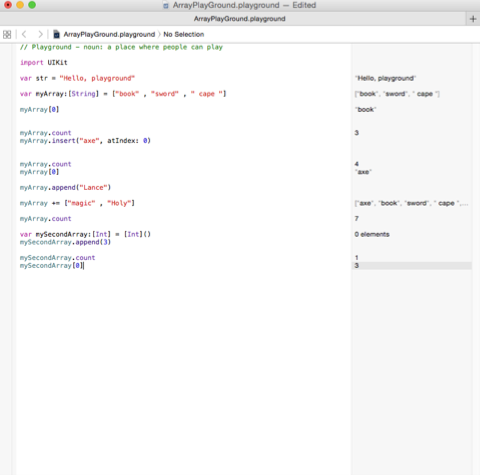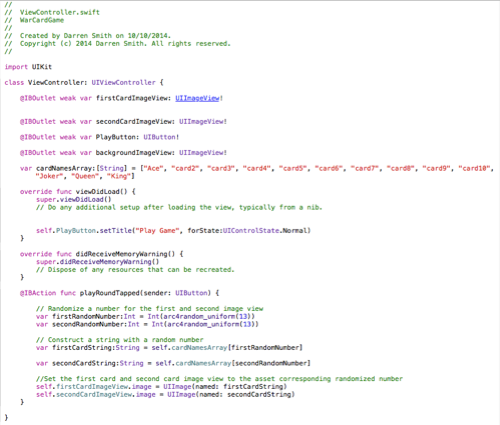# Xcode Swift Code: Introduction to Arrays in Swift Code

Introduction to Arrays

Today is an introduction into Arrays in Xcode 6. In order to learn how created use arrays, I went back to Xcode playground.
An array is used to create a selection of items and array them in a category. In order do this we use the myArray[].

Below in figure 1 is an example of how arrays work.Figure 1:Experimenting with Arrays in Xcode Playground

Now that I understand how the function works, i head back to the card game project in Xcode 6. I then applied the myArray[] function for the UI Image card image underneath the IB Outlet Action button action function. Below in figure 2 is the implementation of the function to the game.Figure 2: Implementing a Card Array that will randomise the Card Selection